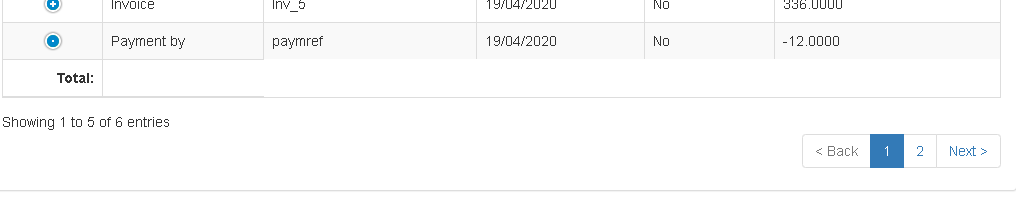# FooterCallback

edited April 2020

Hi
I'm trying to implement this ex. https://datatables.net/examples/advanced_init/footer_callback but can't exactly see what the html should be for my table. Here's the table I have:

``````<table id="tblDataTable1" class="table table-bordered table-striped table-hover" style="width:100%">
<tr>
<th></th>
<th>ID</th>
<th>ContactType</th>
<th>ContactID</th>
<th>Type</th>
<th>Reference</th>
<th>PaymentMethod</th>
<th>Date</th>
<th>Notes</th>
<th>Representative</th>
<th>Paid</th>
<th>AttachedFile</th>
<th>NetAmount</th>
<th>Tax</th>
<th>GrossAmount</th>
</tr>
<tfoot>
<tr>
<th colspan="4" style="text-align:right">Total:</th>
<th></th>
</tr>
</tfoot>
</table>
``````

The data displays fine but if I add the footer I get a fuzzy footer, it does not appear under the table and the sum does not show:Here's my footer js code which I have in the DT's structure:

``````                footerCallback: function ( row, data, start, end, display ) {

var api = this.api(), data;
// Remove the formatting to get integer data for summation
var intVal = function ( i ) {
return typeof i === 'string' ?
i.replace(/[\\$,]/g, '')*1 :
typeof i === 'number' ?
i : 0;
};
// Total over all pages
dblNetTotal = api
.column(12)
.data()
.reduce( function (a, b) {
return intVal(a) + intVal(b);
}, 0 );

// Update footer
\$( api.column(12).footer() ).html(
dblNetTotal
);
},
``````

Thanks.

Edited by Kevin:  Syntax highlighting. Details on how to highlight code using markdown can be found in this guide

• The data displays fine but if I add the footer I get a fuzzy footer, it does not appear under the table

Not sure what you mean by fuzzy footer nor that it doesn't appear under the table. Looks like its under the table.

and the sum does not show

Looks like you are using Responsive.

``````    // Update footer
\$( api.column(12).footer() ).html(
dblNetTotal
);
``````

Is column(12) hidden by responsive? Responsive will hide the corresponding footer `th` elements. I'm not sure how well responsive will work with `colspan` in the footer. You will need to experiment with that.

Kevin

• edited April 2020

column 12 is set as shown

• Footer comes up as another row rather than under the table as per the example in the link and the lines of that row seems to have thinned or disappeared altogther, please see screenshot

• I use bootstrap 3

• The lines thin out in the row that holds the footer in my screenshot

The footer will change along with responsive. Without seeing specifically what you have its hard to say what to change. See this example using a footer with BS4 and Responsive:

Make changes to it to see how the footer behaves when the columns change.

the footer shows as anohter row rather than a footer under the table.

You can use CSS to apply the styling you want to the footer.

Kevin

• Got i to work. The issue was the colspan. Many thanks.

• edited April 2020

Hi,
One more thing plz: I need to show the footer on another line than the 1st line. See below:

`<tfoot>` `<tr>` `<th colspan="11" style="text-align:right">` `Total1:` `</th>` `<th colspan="3" style="text-align:right">` `<select class="form-control" name="selSome"` `id="selSome" >` `</select>` `</th>` `</tr>` `<tr>` `<th colspan="11" style="text-align:right">` `Total2:` `</th>` `<th colspan="3" style="text-align:right">` `Show footer here` `</th>` `</tr>` `</tfoot>`

How do I set the row I need the footer on in the footerCallback? Thanks

• I'm following the example given here and selectors https://datatables.net/forums/discussion/comment/92486/#Comment_92486

Heres my code:

` footerCallback: function ( row, data, start, end, display ) { //HERE

``````                var api1 = this.api(), data;
var api2 = this.api(), data;
var api3 = this.api(), data;
// Remove the formatting to get integer data for summation
var intVal1 = function ( i ) {
return typeof i === 'string' ?
i.replace(/[\\$,]/g, '')*1 :
typeof i === 'number' ?
i : 0;
};
var intVal2 = function ( i ) {
return typeof i === 'string' ?
i.replace(/[\\$,]/g, '')*1 :
typeof i === 'number' ?
i : 0;
};
var intVal3 = function ( i ) {
return typeof i === 'string' ?
i.replace(/[\\$,]/g, '')*1 :
typeof i === 'number' ?
i : 0;
};
// Total over all pages
dblNetTotal = api1
.column(12)
.data()
.reduce( function (a, b) {
return intVal1(a) + intVal1(b);
}, 0 );
dblTaxTotal = api2
.column(13)
.data()
.reduce( function (a, b) {
return intVal2(a) + intVal2(b);
}, 0 );
dblGroTotal = api3
.column(14)
.data()
.reduce( function (a, b) {
return intVal3(a) + intVal3(b);
}, 0 );

// Update footer
\$('tr:eq(0) th:eq(1)', api1.table().footer()).html(ManageDecimals(4, dblNetTotal));
\$('tr:eq(1) th:eq(3)', api2.table().footer()).html(ManageDecimals(4, dblTaxTotal));
\$('tr:eq(2) th:eq(5)', api3.table().footer()).html(ManageDecimals(4, dblGroTotal));
},`
``````

The footer has 3 tr rows and each row has 2 th's. I'm trying to show dblNetTotal dblTaxTotal dblGroTotal on each one of these lines. 1st th holds a label, 2nd th holds these values. At the moment, I'm only getting dblNetTotal showing. What am I doing wrong? Thanks.

• edited April 2020

th:eq(3)

This would be the 4th `th` in the row. If you have 2 then you will want to use `th:eq(1)` for each row. If this doesn't help please build a simple test case so we can help debug.

BTW you don't need to get multiple instances of the API. You can use just one.

Also use 3 back ticks (```) on separate lines for multi line code.

Kevin

• That did it. Thanks a lot